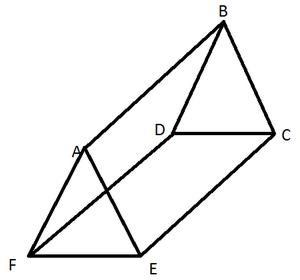Courses

# Test: Visualising Solid Shapes - 1

## 20 Questions MCQ Test Mathematics (Maths) Class 7 | Test: Visualising Solid Shapes - 1

Description
This mock test of Test: Visualising Solid Shapes - 1 for Class 7 helps you for every Class 7 entrance exam. This contains 20 Multiple Choice Questions for Class 7 Test: Visualising Solid Shapes - 1 (mcq) to study with solutions a complete question bank. The solved questions answers in this Test: Visualising Solid Shapes - 1 quiz give you a good mix of easy questions and tough questions. Class 7 students definitely take this Test: Visualising Solid Shapes - 1 exercise for a better result in the exam. You can find other Test: Visualising Solid Shapes - 1 extra questions, long questions & short questions for Class 7 on EduRev as well by searching above.
QUESTION: 1

### The number of faces of a sphere is _______.

Solution:

Sphere has only one face which is the only circular surface

QUESTION: 2

Solution:
QUESTION: 3

### The number of vertices of a cone is _______.

Solution:
QUESTION: 4

The number of faces of a triangular prism is _______.

Solution:

A triangular prism has 2 triangles and 3 rectangles. The one with 4 faces is triangular pyramid.

QUESTION: 5

The number of faces of a square pyramid is _______.

Solution:
QUESTION: 6

The number of faces of a triangular pyramid or tetrahedron is _______.

Solution:
QUESTION: 7

The number of triangular faces of a triangular prism is _______.

Solution:QUESTION: 8

The number of rectangular faces of a triangular prism is _______.

Solution:

A triangular prism has 3 rectangular faces joining the two triangles.

QUESTION: 9

The number of triangular faces of a rectangular pyramid is _______.

Solution:
QUESTION: 10

Which of the following is the number of vertices of sphere?

Solution:
QUESTION: 11

The number of edges of a triangular prism is _______.

Solution:
QUESTION: 12

The number of edges of a square pyramid is _______.

Solution:

The square base has 4 edges and all the vertices joining one vertex at the top form 4 more edges which totals to 8.

QUESTION: 13

The number of edges of a triangular pyramid is _______.

Solution:
QUESTION: 14

The number of edges of a rectangular pyramid is _______.

Solution:
QUESTION: 15

The number of faces of a triangular prism is _______.

Solution:

A triangular prism consists of 2 triangles and 3 rectangles. So it has two triangular faces and three rectangular faces. So it has 5 faces.

QUESTION: 16

The number of faces of a triangular pyramid is _______.

Solution:
QUESTION: 17

The number of faces of a square pyramid is _______.

Solution:
QUESTION: 18

The number of faces of a rectangular prism is _______.

Solution:
QUESTION: 19

The corners of a solid shape are called its ________.

Solution:

The corners of a solid shape are called its vertices; the line segments of itsskeleton are its edges; and its flat surfaces are its faces.

QUESTION: 20

A _______ is a skeleton-outline of a solid that can be folded to make it

Solution: